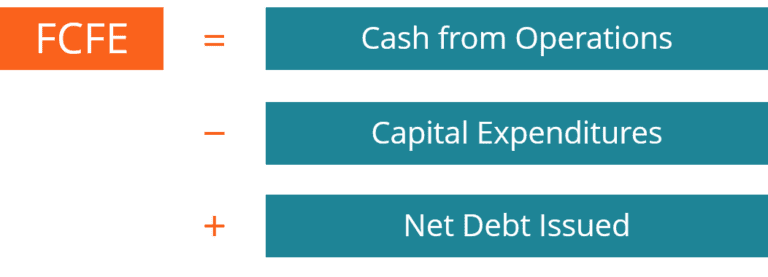## Free Cashflow to Equity Model (FCFE): Equity Valuation

The purpose of equity valuation is to understand and evaluate the intrinsic value of a company which is listed on the public market. A good point to understand is the difference between the book value of assets, liabilities or equity and the market value of the same. The book value is the value at which the asset was initially purchased for; similarly for equities, the book value represents the shareholder’s equity as is listed on a firm’s balance sheet. The market value on the other hand is the total market capitalization of the firm which is calculated by multiplying the price of the share in the public market’s stock exchanges into the total number of shares outstanding of the firm. In the case of liabilities, it is a challenge to obtain the market value of bonds for instance, because each debt security is unique, and it can be difficult to value a security which is not traded at high volumes; therefore, many analysts choose to use the book value of the debt security as the market value. The more divergence of a security’s market price from its book value, the more the security is mis-priced, and the more opportunity exists for traders, speculators, and investors to take advantage of this mispricing and enjoy profits by either buying, selling, or holding.There is a need for this valuation because in today’s financial markets, there are more than billions of shares1 are traded each day all over the US and even more in the world. These large transactions cause the price of securities and financial assets to fluctuate. Equity valuation helps to understand the effects and the status of such mispricing to help market forces interact and return prices to the equilibrium level.

There are various methods of conducting equity valuation, it depends on the perspective of the valuation one is applying. The most commonly used methods include present value models, multiplier models and asset-based models.

Present Value Models:

Present value models are based on the going business concern of firms which believe that the firm will go on forever and create cashflows. Therefore, there is a necessity to assume a future value of cashflows and get it back to match the equivalent value of itself today. This is done by discounting those cashflows using an interest rate which is also assumed as rates can change in the future. The growth rate of these cashflows is also assumed based on trends observed and the prospective future actions of the firm.

Discounted Cash Flow Model:

Free Cashflow to Equity Model (FCFE): This model is used when an analyst wants to value the firm from the perspective of a majority shareholder’s perspective; this means that the perspective is from the point of view that more than 50% of the firm’s equity is owned. Therefore, we use the free cash flow of the firm. These cashflows can either be viewed as the free cashflow which a firm earns or the cashflow that remains with the firm before paying the taxes and interest. The first step is to take the EBIT (Earnings before interest and taxes), also known as the operating profit which is obtained by subtracting the cost of goods sold and operating expenses from the sales revenue, and then understanding the operating cashflow (OCF) of the firm. In order to reach the OCF, we add back depreciation to the EBIT, this is because the depreciation is a non-cash item which is subtracted in the income statement, deduct taxes and add the change in net working capital (current assets minus current liabilities from the balance sheet). Once arrived at the OCF, the FCFE or the Free cash flow to Equity can be calculated by adding the net borrowing and subtracting the Fixed Capital Investment (found on the statement of cashflows).

The formulas are as follows:

EBIT = Revenues – COGS (Cost of Goods Sold) – Operating Expenses

OCF = EBIT + Depreciation – Taxes + Change in Net Working Capital

FCFE = OCF + Net Borrowing – Fixed Capital Investment

Once we arrive at the FCFE, we can use our assumed growth rates to calculate the firm’s future cash flows. The future cash flows of the firm discounted back are known as the terminal value, which can also be shown on the excel snapshot below. The entire process of obtaining the FCFE is also explained. In the example, the terminal value is \$2406, which is then discounted back along with the cash flows of each year of the forecast before the terminal value. All these cash flows are discounted back using an interest rate or the ‘Cost of Capital’, which is 7% in the top segment of the example. Once discounted back, the total firm equity value is calculated by adding all the present value of the cash flows, and in the example, this number is \$2016.1. The last step is to divide this total equity value by the total number of outstanding shares of the firm in the open market to get the intrinsic value of each share. This share price is then compared with the actual share price to check whether the stock is overvalued, undervalued, or fairly valued. The stock is undervalued if the intrinsic value is greater than the current share price in the market and vice versa.

The analysis is thoroughly dependent on the growth rate and the cost of capital/interest rate or the discount rate. It can also be calculated using a mathematical formula as follows:

Since, most of these assumptions are subject to uncertainty, analysts are recommended to perform sensitivity analysis using simulations or scenario analysis to see the changes in equity value by changing variables; this helps to provide a range which is a more probable prediction instead of a single value.### Get free consultation from our tech experts## Related articles you may would like to read

### FREE eBOOK: Recommended RFP process for selecting cloud provider

We are here to simply your migration to cloud by embarking on a systematic, planned, stage-wise journey to the cloud.

##### Categories
###### Resources